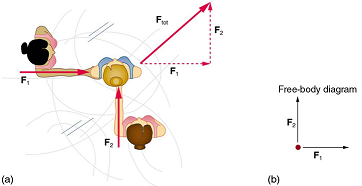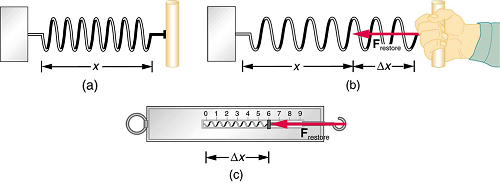Skip to main content

# 7.8: Development of Force Concept

Dynamics is the study of the forces that cause objects and systems to move. To understand this, we need a working definition of force. Our intuitive definition of force—that is, a push or a pull—is a good place to start. We know that a push or pull has both magnitude and direction (therefore, it is a vector quantity) and can vary considerably in each regard. For example, a cannon exerts a strong force on a cannonball that is launched into the air. In contrast, Earth exerts only a tiny downward pull on a flea. Our everyday experiences also give us a good idea of how multiple forces add. If two people push in different directions on a third person, as illustrated in Figure $$\PageIndex{1}$$, we might expect the total force to be in the direction shown. Since force is a vector, it adds just like other vectors, as illustrated in Figure $$\PageIndex{1a}$$ for two ice skaters. Forces, like other vectors, are represented by arrows and can be added using the familiar head-to-tail method or by trigonometric methods. These ideas were developed in Two-Dimensional Kinematics.Figure $$\PageIndex{1}$$: Part (a) shows an overhead view of two ice skaters pushing on a third. Forces are vectors and add like other vectors, so the total force on the third skater is in the direction shown. In part (b), we see a free-body diagram representing the forces acting on the third skater.

Figure $$\PageIndex{1b}$$ is our first example of a free-body diagram, which is a technique used to illustrate all the external forces acting on a body. The body is represented by a single isolated point (or free body), and only those forces acting on the body from the outside (external forces) are shown. (These forces are the only ones shown, because only external forces acting on the body affect its motion. We can ignore any internal forces within the body.) Free-body diagrams are very useful in analyzing forces acting on a system and are employed extensively in the study and application of Newton’s laws of motion.

A more quantitative definition of force can be based on some standard force, just as distance is measured in units relative to a standard distance. One possibility is to stretch a spring a certain fixed distance, as illustrated in Figure $$\PageIndex{2}$$, and use the force it exerts to pull itself back to its relaxed shape—called a restoring force—as a standard. The magnitude of all other forces can be stated as multiples of this standard unit of force. Many other possibilities exist for standard forces. (One that we will encounter in Magnetism is the magnetic force between two wires carrying electric current.) Some alternative definitions of force will be given later in this chapter.Figure $$\PageIndex{2}$$:The force exerted by a stretched spring can be used as a standard unit of force. (a) This spring has a length x when undistorted. (b) When stretched a distance $$\Delta x$$ the spring exerts a restoring force, $$F_{restore}$$ which is reproducible. (c) A spring scale is one device that uses a spring to measure force. The force $$F_{restore}$$ is exerted on whatever is attached to the hook. Here $$F_{restore}$$ has a magnitude of 6 units in the force standard being employed.

TAKE HOME EXPERIMENT: FORCE STANDARDS

To investigate force standards and cause and effect, get two identical rubber bands. Hang one rubber band vertically on a hook. Find a small household item that could be attached to the rubber band using a paper clip, and use this item as a weight to investigate the stretch of the rubber band. Measure the amount of stretch produced in the rubber band with one, two, and four of these (identical) items suspended from the rubber band. What is the relationship between the number of items and the amount of stretch? How large a stretch would you expect for the same number of items suspended from two rubber bands? What happens to the amount of stretch of the rubber band (with the weights attached) if the weights are also pushed to the side with a pencil?

### Summary

• Dynamics is the study of how forces affect the motion of objects.
• Force is a push or pull that can be defined in terms of various standards, and it is a vector having both magnitude and direction.
• External forces are any outside forces that act on a body. A free-body diagram is a drawing of all external forces acting on a body.

## Glossary

dynamics
the study of how forces affect the motion of objects and systems
external force
a force acting on an object or system that originates outside of the object or system
free-body diagram
a sketch showing all of the external forces acting on an object or system; the system is represented by a dot, and the forces are represented by vectors extending outward from the dot
force
a push or pull on an object with a specific magnitude and direction; can be represented by vectors; can be expressed as a multiple of a standard force

## Contributors and Attributions

• Paul Peter Urone (Professor Emeritus at California State University, Sacramento) and Roger Hinrichs (State University of New York, College at Oswego) with Contributing Authors: Kim Dirks (University of Auckland) and Manjula Sharma (University of Sydney). This work is licensed by OpenStax University Physics under a Creative Commons Attribution License (by 4.0).

• Was this article helpful?